# 深入理解K-Means聚类算法

## 概述

### 不同的簇类型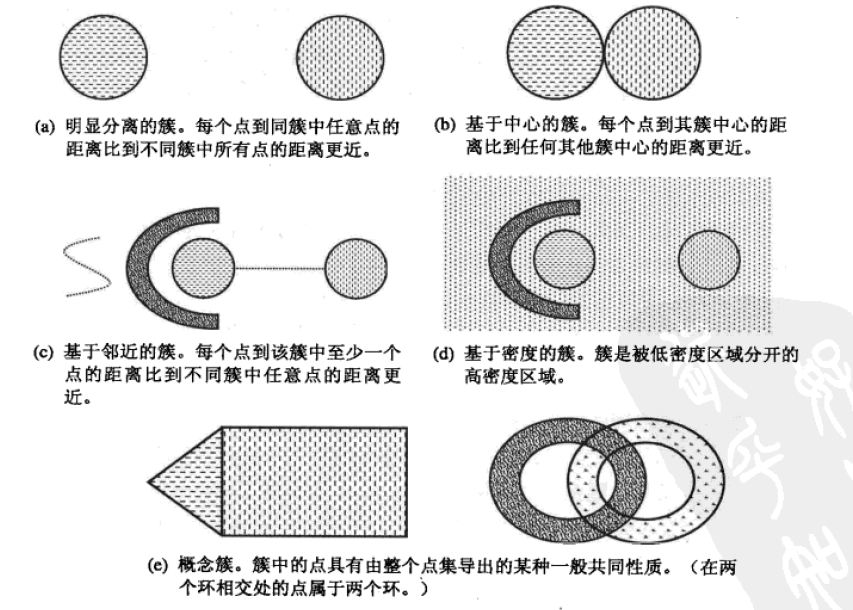1. K均值：

2. 凝聚的层次距离：

3. DBSCAN:

### 距离量度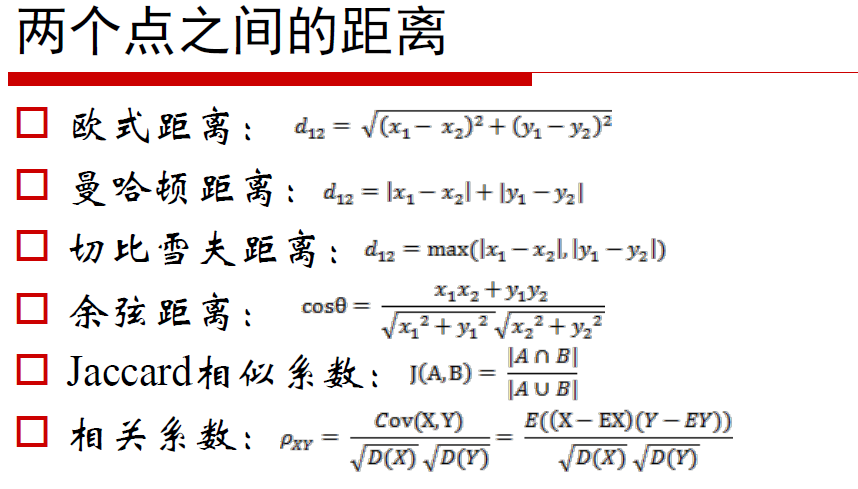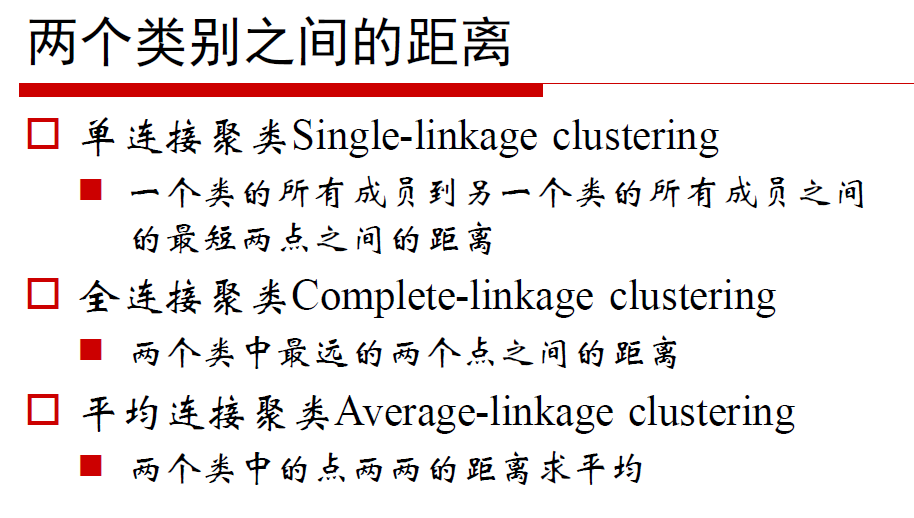## K-Means算法

选择K个点作为初始质心
repeat
将每个点指派到最近的质心，形成K个簇
重新计算每个簇的质心
until 簇不发生变化或达到最大迭代次数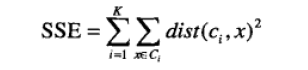k表示k个聚类中心，ci表示第几个中心，dist表示的是欧几里得距离。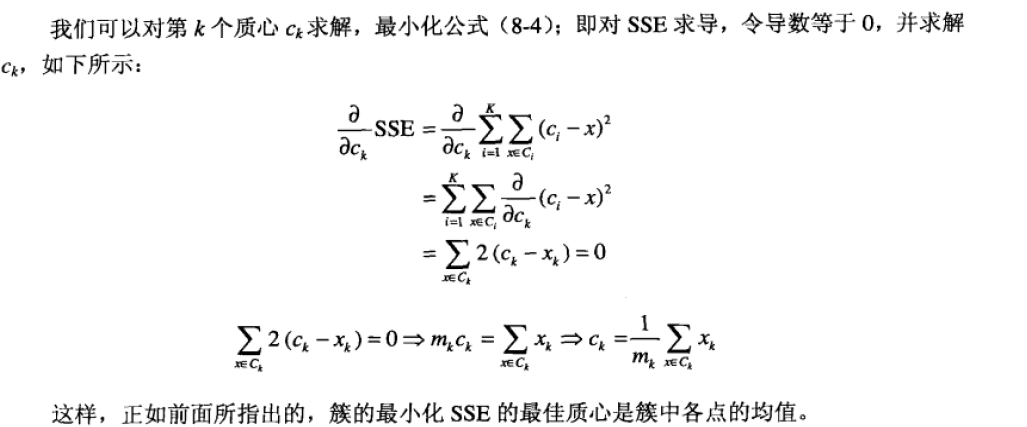# dataSet样本点,k 簇的个数
# disMeas距离量度，默认为欧几里得距离
# createCent,初始点的选取
def kMeans(dataSet, k, distMeas=distEclud, createCent=randCent):
m = shape(dataSet) #样本数
clusterAssment = mat(zeros((m,2))) #m*2的矩阵
centroids = createCent(dataSet, k) #初始化k个中心
clusterChanged = True
while clusterChanged:      #当聚类不再变化
clusterChanged = False
for i in range(m):
minDist = inf; minIndex = -1
for j in range(k): #找到最近的质心
distJI = distMeas(centroids[j,:],dataSet[i,:])
if distJI < minDist:
minDist = distJI; minIndex = j
if clusterAssment[i,0] != minIndex: clusterChanged = True
# 第1列为所属质心，第2列为距离
clusterAssment[i,:] = minIndex,minDist**2
print centroids

# 更改质心位置
for cent in range(k):
ptsInClust = dataSet[nonzero(clusterAssment[:,0].A==cent)]
centroids[cent,:] = mean(ptsInClust, axis=0)
return centroids, clusterAssment

  for cent in range(k):
ptsInClust = dataSet[nonzero(clusterAssment[:,0].A==cent)]
centroids[cent,:] = mean(ptsInClust, axis=0) 

nonzero()返回的是一个二维的数组，其表示非0的元素位置。

>>> from numpy import *
>>> a=array([[1,0,0],[0,1,2],[2,0,0]])
>>> a
array([[1, 0, 0],
[0, 1, 2],
[2, 0, 0]])
>>> nonzero(a)
(array([0, 1, 1, 2]), array([0, 1, 2, 0]))

ptsInClust = dataSet[nonzero(clusterAssment[:,0].A==cent)]

def loadDataSet(fileName):      #general function to parse tab -delimited floats
dataMat = []                #assume last column is target value
fr = open(fileName)
for line in fr.readlines():
curLine = line.strip().split('\t')
fltLine = map(float,curLine) #map all elements to float()
dataMat.append(fltLine)
return dataMat

def distEclud(vecA, vecB):
return sqrt(sum(power(vecA - vecB, 2))) #la.norm(vecA-vecB)

def randCent(dataSet, k):
n = shape(dataSet)
centroids = mat(zeros((k,n)))#create centroid mat
for j in range(n):#create random cluster centers, within bounds of each dimension
minJ = min(dataSet[:,j])
rangeJ = float(max(dataSet[:,j]) - minJ)
centroids[:,j] = mat(minJ + rangeJ * random.rand(k,1))
return centroids

### 运行和结果

>>> from numpy import *
>>> import kMeans
>>> center，clust=kMeans.kMeans(dat,4)
[[ 0.90796996  5.05836784]
[-2.88425582  0.01687006]
[-3.3447423  -1.01730512]
[-0.32810867  0.48063528]]
[[ 1.90508653  3.530091  ]
[-3.00984169  2.66771831]
[-3.38237045 -2.9473363 ]
[ 2.22463036 -1.37361589]]
[[ 2.54391447  3.21299611]
[-2.46154315  2.78737555]
[-3.38237045 -2.9473363 ]
[ 2.8692781  -2.54779119]]
[[ 2.6265299   3.10868015]
[-2.46154315  2.78737555]
[-3.38237045 -2.9473363 ]
[ 2.80293085 -2.7315146 ]]
# 作图
>>>kMeans(dat,center)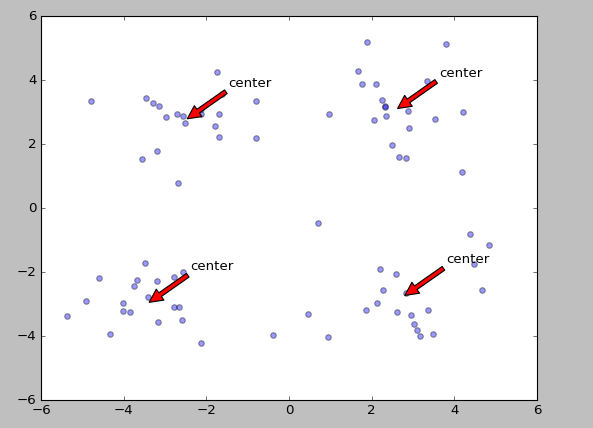def draw(data,center):
length=len(center)
fig=plt.figure
# 绘制原始数据的散点图
plt.scatter(data[:,0],data[:,1],s=25,alpha=0.4)
# 绘制簇的质心点
for i in range(length):
plt.annotate('center',xy=(center[i,0],center[i,1]),xytext=\
(center[i,0]+1,center[i,1]+1),arrowprops=dict(facecolor='red'))
plt.show()

### K-Means算法的缺陷

k均值算法非常简单且使用广泛，但是其有主要的两个缺陷：
1. K值需要预先给定，属于预先知识，很多情况下K值的估计是非常困难的，对于像计算全部微信用户的交往圈这样的场景就完全的没办法用K-Means进行。对于可以确定K值不会太大但不明确精确的K值的场景，可以进行迭代运算，然后找出Cost Function最小时所对应的K值，这个值往往能较好的描述有多少个簇类。
2. K-Means算法对初始选取的聚类中心点是敏感的，不同的随机种子点得到的聚类结果完全不同
3. K均值算法并不是很所有的数据类型。它不能处理非球形簇、不同尺寸和不同密度的簇，银冠指定足够大的簇的个数是他通常可以发现纯子簇。
4. 对离群点的数据进行聚类时，K均值也有问题，这种情况下，离群点检测和删除有很大的帮助。

#### 拙劣的初始质心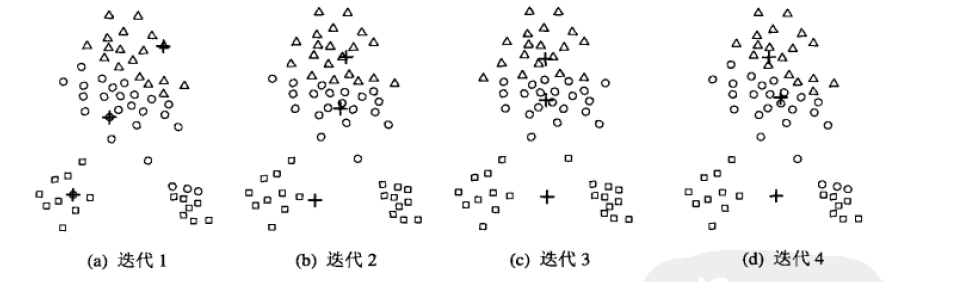## K-Means优化算法

### bisecting K-means

将所有的点看成是一个簇

对于每一个簇
计算总误差
在给定的簇上进行K-均值聚类,k值为2
计算将该簇划分成两个簇后总误差
选择是的误差最小的那个簇进行划分

def biKmeans(dataSet, k, distMeas=distEclud):
m = shape(dataSet)
# 这里第一列为类别，第二列为SSE
clusterAssment = mat(zeros((m,2)))
# 看成一个簇是的质心
centroid0 = mean(dataSet, axis=0).tolist()
centList =[centroid0] #create a list with one centroid
for j in range(m):    #计算只有一个簇是的误差
clusterAssment[j,1] = distMeas(mat(centroid0), dataSet[j,:])**2

# 核心代码
while (len(centList) < k):
lowestSSE = inf
# 对于每一个质心，尝试的进行划分
for i in range(len(centList)):
# 得到属于该质心的数据
ptsInCurrCluster =\ dataSet[nonzero(clusterAssment[:,0].A==i),:]
# 对该质心划分成两类
centroidMat, splitClustAss = kMeans(ptsInCurrCluster, 2, distMeas)
# 计算该簇划分后的SSE
sseSplit = sum(splitClustAss[:,1])
# 没有参与划分的簇的SSE
sseNotSplit = sum(clusterAssment[nonzero(clusterAssment[:,0].A!=i),1])
print "sseSplit, and notSplit: ",sseSplit,sseNotSplit
# 寻找最小的SSE进行划分
# 即对哪一个簇进行划分后SSE最小
if (sseSplit + sseNotSplit) < lowestSSE:
bestCentToSplit = i
bestNewCents = centroidMat
bestClustAss = splitClustAss.copy()
lowestSSE = sseSplit + sseNotSplit

# 较难理解的部分
bestClustAss[nonzero(bestClustAss[:,0].A == 1),0] = len(centList) #change 1 to 3,4, or whatever
bestClustAss[nonzero(bestClustAss[:,0].A == 0),0] = bestCentToSplit
print 'the bestCentToSplit is: ',bestCentToSplit
print 'the len of bestClustAss is: ', len(bestClustAss)
centList[bestCentToSplit] = bestNewCents[0,:].tolist()#replace a centroid with two best centroids
centList.append(bestNewCents[1,:].tolist())
clusterAssment[nonzero(clusterAssment[:,0].A == bestCentToSplit),:]= bestClustAss#reassign new clusters, and SSE
return mat(centList), clusterAssment

      bestClustAss[nonzero(bestClustAss[:,0].A == 1),0] = len(centList) #change 1 to 3,4, or whatever
bestClustAss[nonzero(bestClustAss[:,0].A == 0),0] = bestCentToSplit

  centList[bestCentToSplit] = bestNewCents[0,:].tolist()#replace a centroid with two best centroids
centList.append(bestNewCents[1,:].tolist())
clusterAssment[nonzero(clusterAssment[:,0].A == bestCentToSplit),:]= bestClustAss#reassign new clusters, and SSE

#### 运行与结果

>>> from numpy import *
>>> import kMeans
>>> dat = mat(kMeans.loadDataSet('testSet2.txt'))
>>> cent,assment=kMeans.biKmeans(dat,3)
sseSplit, and notSplit:  570.722757425 0.0
the bestCentToSplit is:  0
the len of bestClustAss is:  60
sseSplit, and notSplit:  68.6865481262 38.0629506357
sseSplit, and notSplit:  22.9717718963 532.659806789
the bestCentToSplit is:  0
the len of bestClustAss is:  40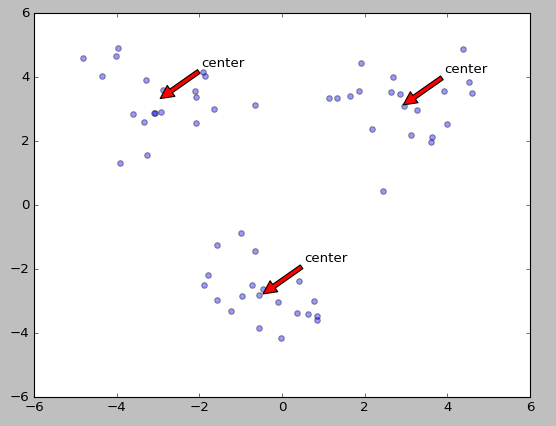#### Mini Batch k-Means

Mini Batch的好处：不必使用所有的数据样本，而是从不同类别的样本中抽取一部分样本来代表各自类型进行计算。n 由于计算样本量少，所以会相应的减少运行时间n 但另一方面抽样也必然会带来准确度的下降。